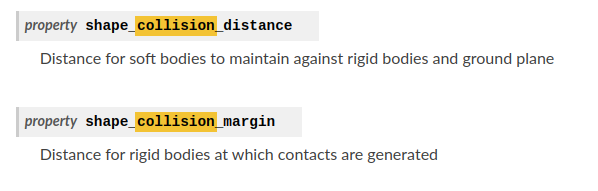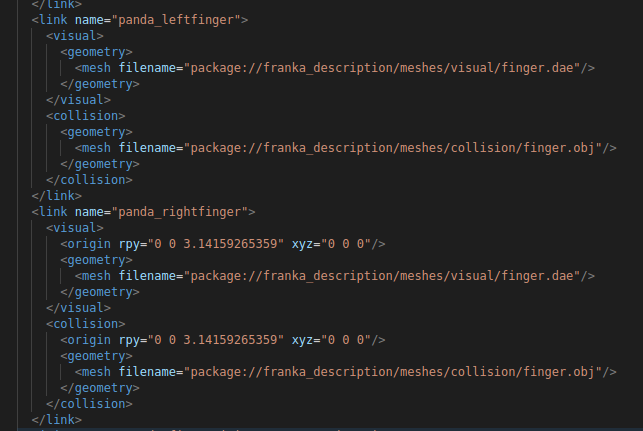# How to enforce self-collisions with Franka?

I am using Isaac Gym and `setup.py` says it is version `1.0.preview4`. I am wondering how to enforce self-collisions for the Franka robot?

In particular, I am running the Franka OSC example from `isaacgym/python/examples/franka_osc.py` and if I change the way that the Franka hand moves, I observe that self-collisions are not enforced correctly. One way to do this is to change this:

``````    # Set desired hand positions
if args.pos_control:
pos_des[:, 0] = init_pos[:, 0] - 0.1
pos_des[:, 1] = math.sin(itr / 50) * 0.2
pos_des[:, 2] = init_pos[:, 2] + math.cos(itr / 50) * 0.2
``````

To this, where instead of subtracting 0.1, I subtract 0.5:

``````    # Set desired hand positions
if args.pos_control:
pos_des[:, 0] = init_pos[:, 0] - 0.5
pos_des[:, 1] = math.sin(itr / 50) * 0.2
pos_des[:, 2] = init_pos[:, 2] + math.cos(itr / 50) * 0.2
``````

This results in a video that looks like this:

Which shows that the Franka gripper is intersecting with other links of the Franka. I am curious if there is a way to fix this? For example do I have to adjust some simulation parameters like this in the docs?Or is there a way to fix this with the URDF file of the Franka? But the Franka URDF shows, for example, that `panda_leftfinger` and `panda_rightfinger` already have collision geometries with `<collision> ... </collision>` there.I figured out the solution, it was to change:

`franka_handle = gym.create_actor(env, franka_asset, pose, "franka", i, 1)`

to

`franka_handle = gym.create_actor(env, franka_asset, pose, "franka", i, 0)`

From the documentation this is changing the `param6` to be 0 so items in the same collision group will NOT mask off collision (which I interpret as, it will ENABLE self collision).

1 Like

This topic was automatically closed 14 days after the last reply. New replies are no longer allowed.